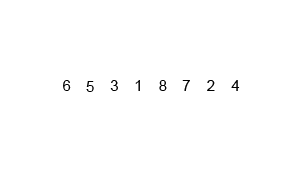## Sunday, 28 December 2014

### C Program for sorting elements using Merge sort

 This a C program which sorts all the elements using Merge Sort Technique. Merge sort is a  divide and conquer technique , here the element list is divided into half and that halves are again divided into halves until only 2 elements left . Then compare those 2 elements , smallest one is kept  first,  repeat the same process until are the halves are sorted , and repeat the same process but in reverse order to unite the element list into one again. See the animation below to understand ,"Merge-sort-example" by Swfung8 - Own work. Licensed under CC BY-SA 3.0 via Wikimedia Commons.

## PROGRAM :

```#include <stdio.h>
#include <stdlib.h>
void mergeSort(int [], int, int, int);
void partition(int [],int, int);
int main()
{
int list;
int i, n;

printf("How many numbers ? \t");
scanf("%d", &n);
printf("\n\n");
for(i = 0; i < n; i++)
{
printf("Enter number %d :\t",i+1);
scanf("%d", &list[i]);
}
partition(list, 0, n - 1);
printf("\n\nAfter merge sort:\n");
for(i = 0;i < n; i++)
{
printf("%d\t",list[i]);
}
printf("\n\n");
return 0;
}
void partition(int list[],int low,int high)
{
int mid;

if(low < high)
{
mid = (low + high) / 2;
partition(list, low, mid);
partition(list, mid + 1, high);
mergeSort(list, low, mid, high);
}
}

void mergeSort(int list[],int low,int mid,int high)
{
int i, midd, k, loww, temp;

loww = low;
i = low;
midd = mid + 1;
while ((loww <= mid) && (midd <= high))
{
if (list[loww] <= list[midd])
{
temp[i] = list[loww];
loww++;
}
else
{
temp[i] = list[midd];
midd++;
}
i++;
}
if (loww > mid)
{
for (k = midd; k <= high; k++)
{
temp[i] = list[k];
i++;
}
}
else
{
for (k = loww; k <= mid; k++)
{
temp[i] = list[k];
i++;
}
}

for (k = low; k <= high; k++)
{
list[k] = temp[k];
}
}
```

## OUTPUT :C Program for implementing MergeSort